Download PresentationDecision support tools

# Decision support tools - PowerPoint PPT Presentation

Decision support tools. Lecture 9: Preparation for Prac 2. Mathematical models. Decision variables Values that you can change E.g. selling price of your products, staff wages Uncontrollable variables Values that you can’t change E.g. rand/dollar exchange rate, income tax payableI am the owner, or an agent authorized to act on behalf of the owner, of the copyrighted work described.
Download Presentation## Decision support tools

An Image/Link below is provided (as is) to download presentation

Download Policy: Content on the Website is provided to you AS IS for your information and personal use and may not be sold / licensed / shared on other websites without getting consent from its author.While downloading, if for some reason you are not able to download a presentation, the publisher may have deleted the file from their server.

- - - - - - - - - - - - - - - - - - - - - - - - - - E N D - - - - - - - - - - - - - - - - - - - - - - - - - -
Presentation Transcript### Decision support tools

Lecture 9: Preparation for Prac2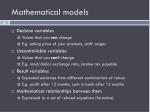Mathematical models
• Decision variables
• Values that you can change
• E.g. selling price of your products, staff wages
• Uncontrollable variables
• Values that you can’t change
• E.g. rand/dollar exchange rate, income tax payable
• Result variables
• Expected outcomes from different combinations of values
• E.g. profit after 12 months, cash in bank after 12 months
• Mathematical relationships between them
• Expressed as a set of related equations (formulas)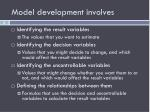Model development involves
• Identifying the result variables
• The values that you want to estimate
• Identifying the decision variables
• Values that you might decide to change, and which would affect the result variables
• Identifying the uncontrollable variables
• Values that might change whether you want them to or not, and which would affect the result variables
• Defining the relationships between them
• Formulas that use the decision and uncontrollable variables to calculate the result variables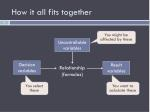How it all fits together

Uncontrollable variables

Decision variables

Result variables

Relationship

(formulas)

You select these

You want to calculate these

You might be affected by these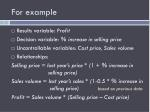For example
• Results variable: Profit
• Decision variable: % increase in selling price
• Uncontrollable variables: Cost price, Sales volume
• Relationships:

Selling price = last year’s price * (1 + % increase in selling price)

Sales volume = last year’s sales * (1-0.5 * % increase in selling price) based on previous data

Profit = Sales volume * (Selling price – Cost price)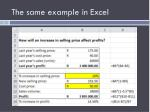Excel tools for decision modelling
• Scenario Manager
• Compares results of different “what-if” scenarios
• Data tables
• Evaluate sensitivity to change
• Goal seek
• Changes only one value to achieve desired outcome
• Solver
• Takes multiple constraints (conditions) into account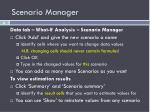Scenario Manager

Data tab – What-If Analysis – Scenario Manager

• Click ‘Add’ and give the new scenario a name
• Identify cells where you want to change data values

N.B. changing cells should never contain formulas!

• Click OK
• Type in the changed values for this scenario
• You can add as many more Scenarios as you want

To view estimation results

• Click ‘Summary’ and ‘Scenario summary’
• Identify the result cells that you want to estimate values for
• You can use ‘Show’ to reinstate values from any scenario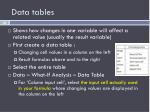Data tables
• Shows how changes in one variable will affect a related value (usually the result variable)
• First create a data table :
• Changing cell values in a column on the left
• Result formulas above and to the right
• Select the entire table
• Data – What-If Analysis – Data Table
• For ‘Column input cell’, select the input cell actually used in your formula whose changing values are displayed in the column on the leftGoal seek
• Calculates the value needed for one specific input cell, to get the desired result from a formula
• Data – What-If Analysis – Goal Seek
• Set cell: cell reference for result variable
• To value: result that you want to get
• By changing cell: cell reference for the input value that should be changed to get the result you want
• OK

12%Solver
• May need to be installed as an Add-In under File – Options
• Select the cell containing the Result formula, and then click on the Solver icon
• A dialog box will open
• Set Target Cell is already defined (default is max)
• By changing cells lists values to be changed
• Constraints define conditions that must be met, e.g. G7 <= 120 where G12 is a calculated value
• Click Solve to calculate the resultPreparation for DST prac 2
• In Prac 2 you will need to be able to:
• Create formulas that reflect business logic correctly
• Calculations involving variables
• Cell referencing (absolute vs relative)
• IF statements
• Use the Scenario Manager
• Create a Data Table
• Perform a Goal Seek